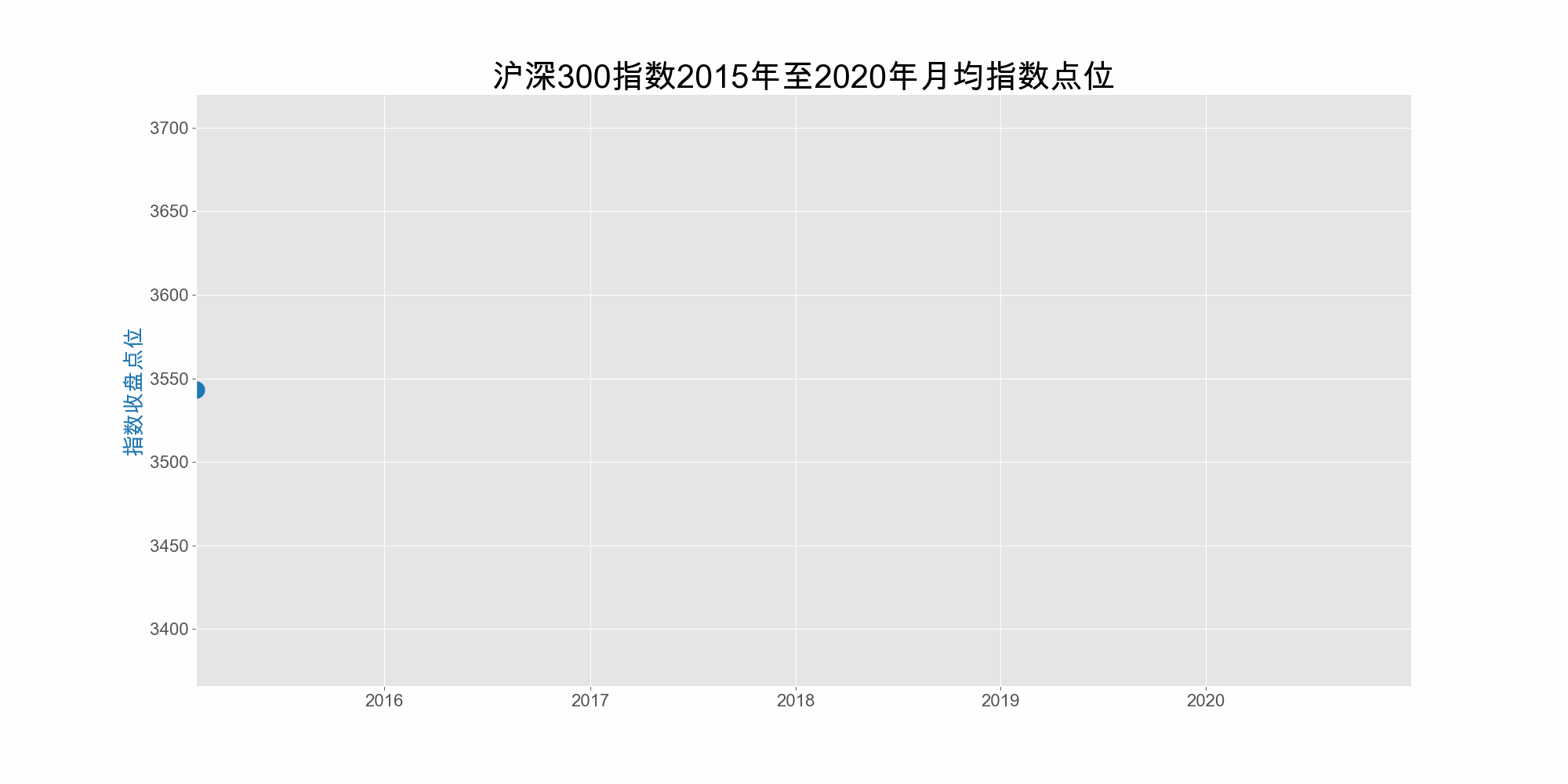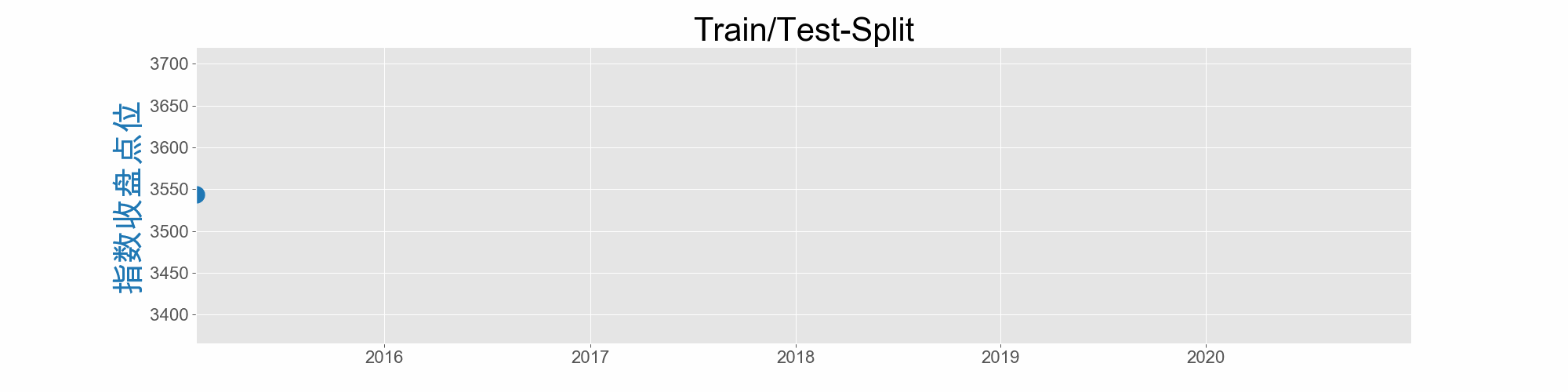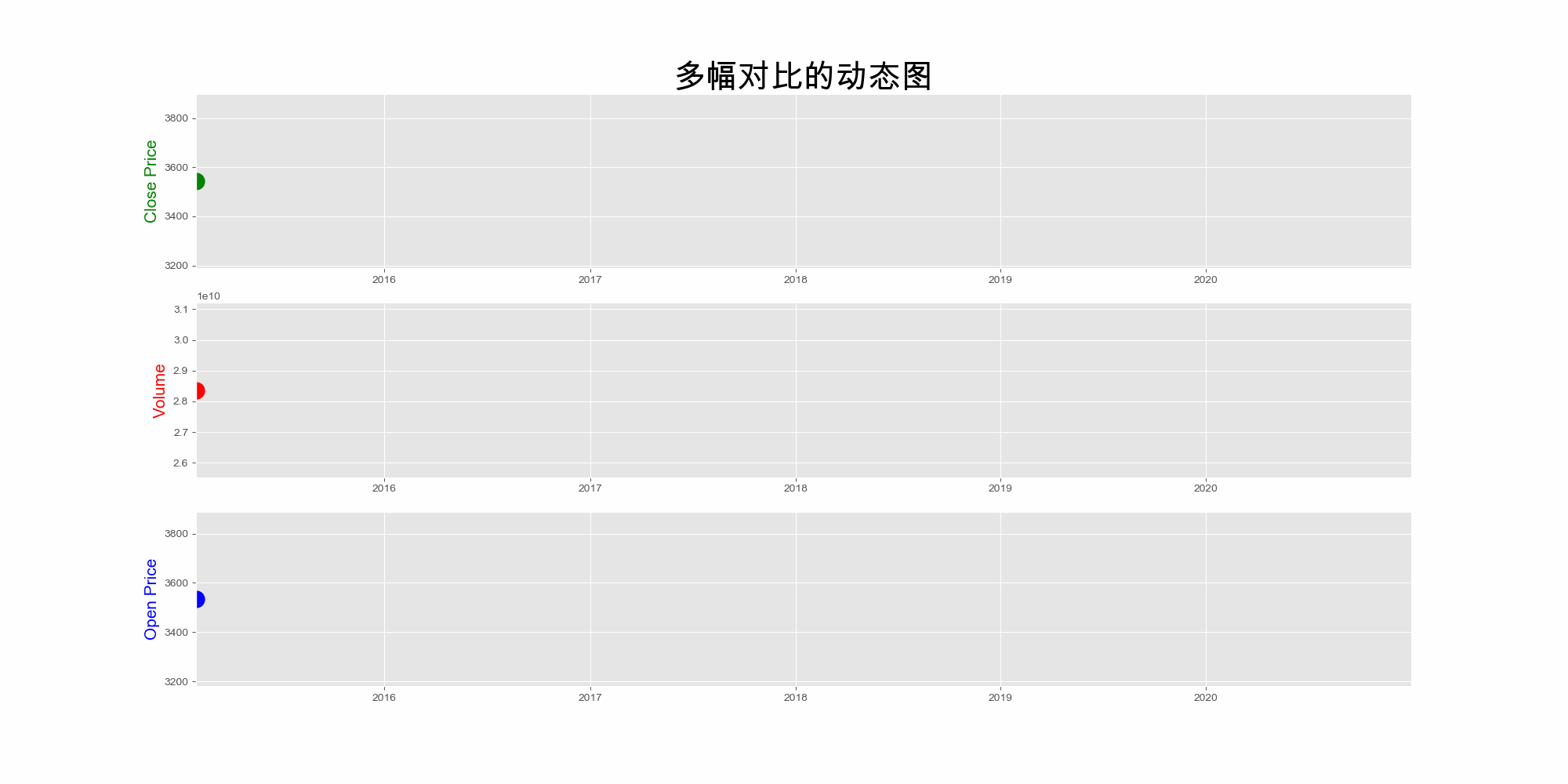Matplotlib

# Matplotlib 动态图还可以这样玩，收藏了！

Matplotlib 是 Python 数据可视化中不可或缺的一个可视化库，「Python数据之道」之前也给大家分享了一系列 Matplotlib 从基础到进阶的内容，包括：

## 4种类型的动态 gif 图

### 单曲线动态图START = df_300_price.index
END = df_300_price.index[-1]

### step1:创建动态图每帧的自定义函数
@gif.frame
def plot(df,date):
df = df.loc[df.index:pd.Timestamp(date)]
fig, (ax1) = plt.subplots(1,figsize=(20,10),dpi=100)
ax1.plot(
df.close, marker='o',
linestyle='--',
linewidth=3,
markersize=15,
color = 'tab:blue'
)
y_max = round(df.close.max()*1.05)
y_min = round(df.close.min()*0.95)
ax1.set_title('沪深300指数2015年至2020年月均指数点位',fontsize=30)
#     ax1.set_title('average close price of Hushen 300 index during 2010 to 2020', fontsize=30)
ax1.set_xlim([START,END])
ax1.set_ylim([y_min,y_max])
ax1.set_ylabel('指数收盘点位', color='tab:blue', fontsize=20)
# 设置刻度字体大小
ax1.tick_params(labelsize=16)

### setp2:将动画帧组合起来
frames = []
for date in pd.date_range(start =START, end=END,freq='1M'):
frame = plot(df_300_price,date)
frames.append(frame)

### step3：使用 gif库 保存图片
date1 = datetime.datetime.now().strftime('%H%M%S')
output1 = "output1_{}.gif".format(date1)
print(output1)
gif.save(frames, output1,duration=0.5,unit = 's')

# step4：在网页中看 gif 图片的效果
HTML('<img src="./{}" />'.format(output1))


### 分段分不同背景颜色的动态图### 双曲线动态图### 多图多曲线动态图### 代码文件获取